3rd Grade Math Lesson on Finding the Area with Decomposition and Addition

Use decomposition of rectilinear figures and addition of the areas of the decomposed figures in the context of solving real world and mathematical problems.

Lesson Objective: The lesson is aligned to the Common Core State Standards for Mathematics – 3.MD.7d Geometric Measurement – Recognize area as additive.

Materials Required: graph paper, calculator

Lesson Procedure: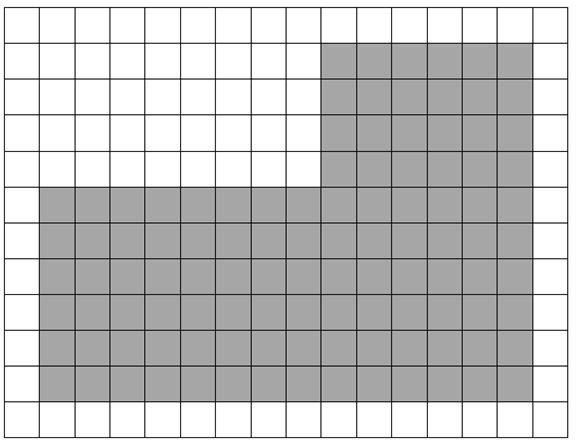Find Area Using Decomposition of Rectilinear Figures and Addition

Kathy wants to install new carpet in her living room. The drawing on the grid below represents the shape of her living room. The length of each side of the squares on the grid measures one foot. What is the total area of Kathy’s living room?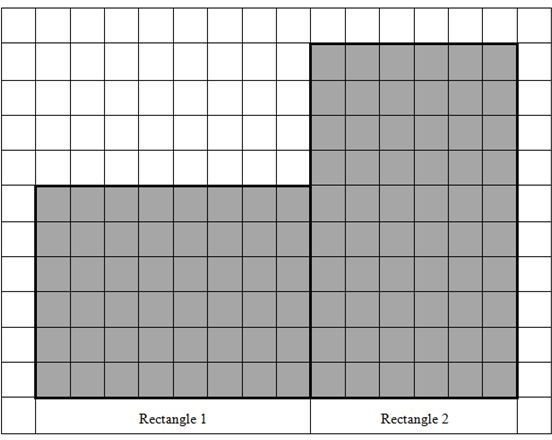1. Decompose the drawing of the living room into two rectangles. Label the rectangles, Rectangle 1 and Rectangle 2.

2. What is the length of the sides of Rectangle 1?

3. What is the length of the sides of Rectangle 2?

4. What is the area of Rectangle 1?

5. What is the area of Rectangle 2?

6. To find the area of Kathy’s living room, add the areas of Rectangle 1 and Rectangle 2.

7. What is the area of Kathy’s living room in square feet?

2. 6 feet and 8 feet

3. 10 feet and 6 feet

4. 48 square feet

5. 60 square feet

6. 48 square feet + 60 square feet

7. 108 square feet

Individual or Group Work: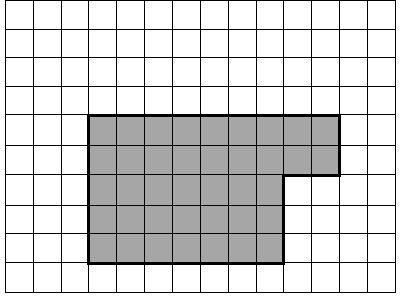Look at the drawings shown on the grid below to help you solve the problems. The length of each side of the squares on the grid measures one foot. To find the total areas, decompose the drawings into rectangles.

1. Find the area of the shaded area on the grid shown below.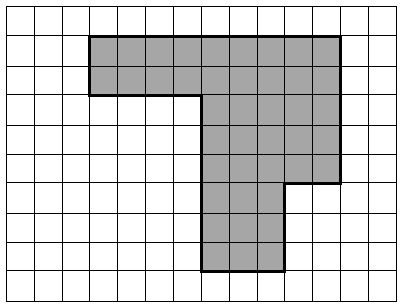2. Find the area of the shaded area on the grid shown below.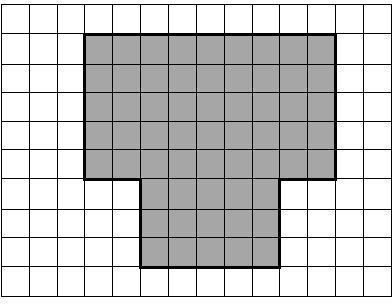3. Rhys wants to plant a garden. The drawing on the grid below represents the shape of the garden he wants to plant. What is the total area of the garden Rhys wants to plant?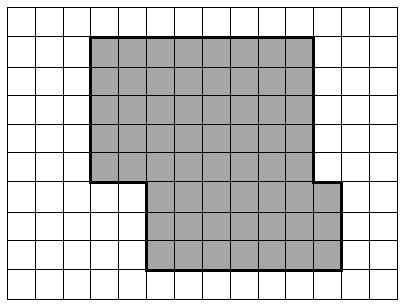4. Jack wants to build a patio in his back yard. The drawing on the grid below represents the shape of the patio he wants to build. What is the total area of the patio Jack wants to build?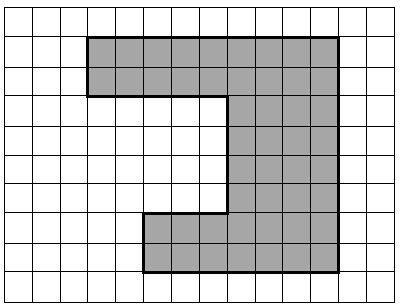5. Jasper wants to build a sidewalk around his patio. The drawing on the grid below represents the shape of the sidewalk he wants to build. What is the total area of the sidewalk Jasper wants to build?

1. 39 square feet

2. 42 square feet

3. 60 square feet

4. 61 square feet

5. 48 square feet